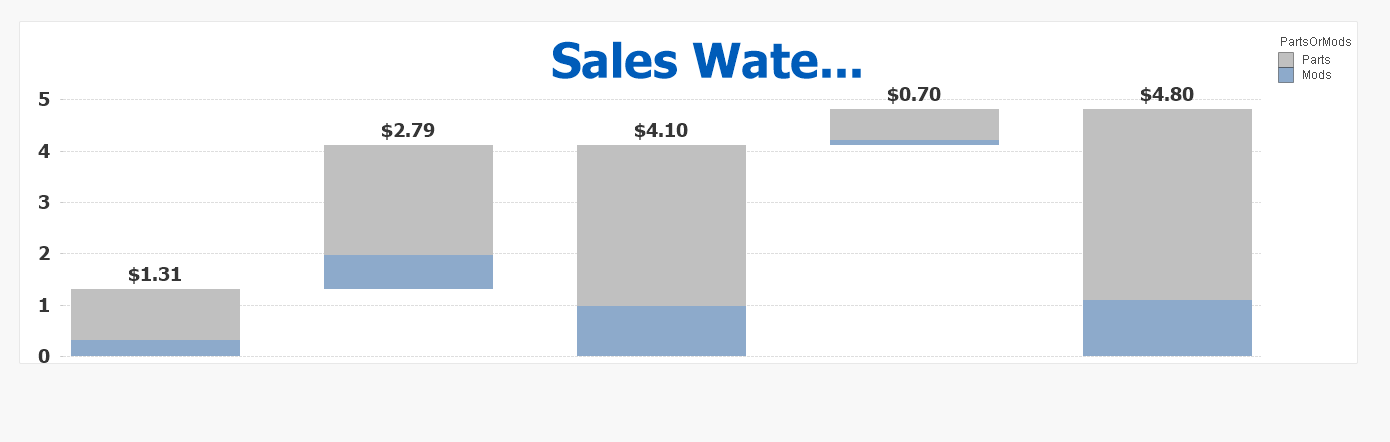QlikView Layout & Visualizations

Discussion Board for collaboration on QlikView Layout & Visualizations.

HighlightedNot applicable

Stacked Waterfall Chart --- w/ .QVW and example

Hi Qlik Community!

I have the following waterfall chart -- but I can't seem to solve the error below. I have my chart broken into two dimensions (Parts/Mods) and when using an offset expression, there is a gap between the two bars in the chart. The arrows specify the direction each "semi stack" should "move" to be located in the correct position.Would really appreciate some help solving this problem! --- QVW and dataset attached.

I tried to use logic similar to the following to solve..but it didn't work (posted so you can see my logic/reasoning)

Example for conv orders offset expression -- granted this is not the true syntax, merely a description of the logic involved.

if both parts and mods are selected (or no selection specified) ----> offset the mods stack by the total backlog, and offset the parts stack by total backlog + mods conv orders

if only parts selected ---> offset by backlog(parts)

if only mods selected ---> offset by backlog(mods)

Tags (4)
1 Solution

Accepted SolutionsMVP

Re: Stacked Waterfall Chart --- w/ .QVW and example

May be this for bar offset

=If(RowNo() = 1,

if(

Valuelist('Backlog','Conv Orders','Sales Est','GAP','Sales IOP','phase 6','phase 7') = 'Conv Orders', (sum(total ConvCurrentYearTotalOpenBL)

)/1000000,

if(sum(SalesIOP) - sum(SalesEst) >0,

if(Valuelist('Backlog','Conv Orders','Sales Est','GAP','Sales IOP','phase 6','phase 7') = 'GAP' ,

sum(total SalesEst)/1000000, 0),

if(Valuelist('Backlog','Conv Orders','Sales Est','GAP','Sales IOP','phase 6','phase 7') = 'GAP', sum(SalesIOP)/1000000,0))), 0)3 RepliesMVP

Re: Stacked Waterfall Chart --- w/ .QVW and example

May be this for bar offset

=If(RowNo() = 1,

if(

Valuelist('Backlog','Conv Orders','Sales Est','GAP','Sales IOP','phase 6','phase 7') = 'Conv Orders', (sum(total ConvCurrentYearTotalOpenBL)

)/1000000,

if(sum(SalesIOP) - sum(SalesEst) >0,

if(Valuelist('Backlog','Conv Orders','Sales Est','GAP','Sales IOP','phase 6','phase 7') = 'GAP' ,

sum(total SalesEst)/1000000, 0),

if(Valuelist('Backlog','Conv Orders','Sales Est','GAP','Sales IOP','phase 6','phase 7') = 'GAP', sum(SalesIOP)/1000000,0))), 0)Valued Contributor

Re: Stacked Waterfall Chart --- w/ .QVW and exampleNot applicable

Re: Stacked Waterfall Chart --- w/ .QVW and example

Hey Sunny,

Thanks for your help! Sorry about the double post.

Sergey also posted a great answer on the linked post (in his response here).

Really appreciate it!

-Brian